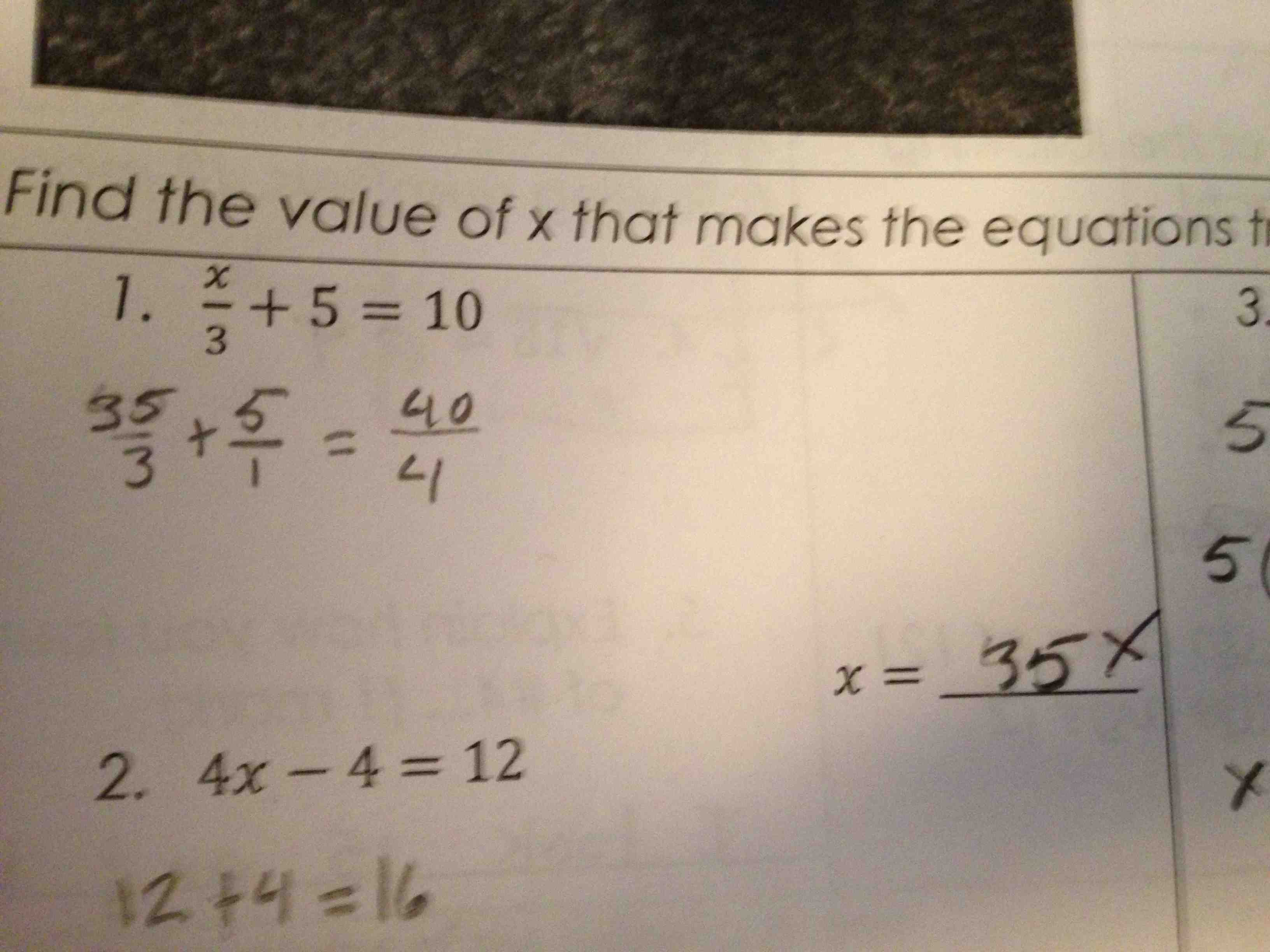Categories

# Fractions and Solving EquationsOffered to you without comment. Say something interesting in the comments.

(Thanks Timon!)

## 8 replies on “Fractions and Solving Equations”

Well, that’s exactly how I’d teach it. If one thing has fractions, make them all fractions, create a common denominator, and now that you have the same “whole”, eliminate it. They started that process… then completely missed the rather important “common denominator” step. Also a bit baffling is the choice of “4” as the right hand side denominator (b/c it’s between 3 and 5??), when “1” was chosen for the 5.

Perversely, I think the core problem is that the student is only seeing ‘individual steps’ as opposed to the equation as a whole. They did step 1, forgot step 2, then solved by inspection. Their response to the question below would back this up, as they’ve written “12+4 = 16”. Not 4x = 16, considering the equation as a whole, only “12+4 = 16”, the ‘first step’ in the process. It wouldn’t surprise me to learn that they then jumped to solving that problem by inspection too.

Of course, I could be way off.

secretseasonssays:

The choice of 4 for the right hand denominator is because it is the sum of the left hand denominators, 3 and 1. If you asked this student what 1/2 + 1/3 is, they would say 2/5, because they are adding numerators and adding denominators.

So here is what I’m pretty confident the student’s thought process was:
(1) x/3 + (wait, let me make 5 into 5/1) = (something)/4 because the denominators add to 4.
(2) OK, now it was supposed to be 10 on the right-hand side so what does the numerator have to be? 40/4 is 10, so ok, 40/4.
(3) OK now looking at the numerators I have x+5=40 so x must be 35.

Can’t believe I didn’t see addition of denominators. Well done.

… and they’re “seeing” steps but not writing things down. What answer was gotten for the next question? There are some pretty interesting intuitions happening here.
The problem on top perfectly follows the “add top and bottom for your answer” rule. So, looks like the student correctly made fractions out of the two known numbers and then made top and bottom work so that they added horizontally.

Do you guys see that erased 4, both behind the 35 in the left fraction, and also in the answer box?

Any ideas what this student was thinking of writing as the answer, originally?

Looks like they wrote 40. I’m thinking maybe they guessed 40 at first. Then they thought about it a bit more, tried to plug in the 40 and saw that it wouldn’t work – even with their incorrect arithmetic. Then they saw 35 would work using their rule of add straight across.

Michellesays:

Student correctly converted 10 to 40/4 and 5 to 5/1. The problem comes with the addition of fractions to solve for x. Student did not solve using common denominator. Student added denominators 3 + 1 = 1 and solve for x by adding numerators 35 + 5 = 40 therefore x = 35. So student convert whole numbers to improper fractions but does not know how to add fractions.

Cynthia Nicolsonsays:

I figure the student’s thinking went something like this: “I’ll leave a blank over the 3 (for the x) plus 5 ..now, what was that rule? I’m supposed to everything into fractions…so I’ll write 5/1 … and if I add those two denominators I get 4 for the denominator on the right. But wait – the right side needs to be equal to 10. Hmmm… I know! – 40 divided by 4 makes 10. Hey – 40 must be the answer! I’ll put 40 above the 3 (where x was supposed to go) and in the answer box. Uh-oh… that doesn’t work. 40 plus 5 isn’t 40…. even I know that… but 35 plus 5 is 40. Erase the 40 … and put in 35 instead.” Something like that.